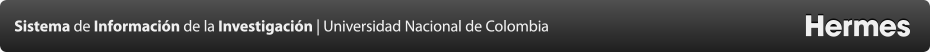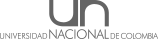Proyectos
A Modified Model to Describe Porosity-Temperature Relationship
Resumen
This work presents a literature review and an analytical model to evaluate the porosity changes due to temperature effect, at which the reservoir rock can be subjected during thermal recovery techniques (i.e., CSS and SAGD). The model is based on two mechanical theories: poroelasticity, which considers three different volumes (bulk, solid frame, and porous) in their answers to stress change, and thermoelasticity that allows calculating the mechanical stresses related to temperature changes using thermal expansion. To achieve a more realistic behavior, a previous model is modified by adding the action of confining pressure as a new variable and multiple modifications of the thermal expansion coefficients from laboratory evidence. With those modifications, problems of either over or underestimation are overcome. In the proposed model, three modifications are applied to achieve a better approach to actual phenomena: 1) The thermal expansion coefficient is understood as no-linear, 2) the expansion of bulk and constitutive material are differentiated, and 3) the bulk volume is dominated by a new concept of modulated strain that combines thermal and confining stress, in that way, the confining stress is included into the model. It is found that porosity¿s changes are result of the interaction between the thermal and confining stress. In that way, the model describes two phases. In the first phase, porosity decrease as a consequence of the constant bulk volume while the solid volume expands accumulating thermal stress. In the second phase, thermal stress overcomes confining one and a porosity recovery happens. Some applications, such as permeability calculation using Carman-Kozeny correlations are presented. These calculations may give an idea on how the temperature could change the formation petrophysical properties. Furthermore, a simple formulation of a safe injection temperature can be derived from the concept of maximum allowed reservoir deformation, which depends on temperature change and overburden pressure. Finally, sensitivities of the new model are presented in order to analyze the impact of mechanical and thermal properties within the porosity calculations.
Convocatoria
 Nombre de la convocatoria: CONVOCATORIA DE APOYO PARA CORRECCIÓN DE ESTILO DE ARTÍCULOS DE INVESTIGACIÓN Modalidad: Modalidad única
Responsable